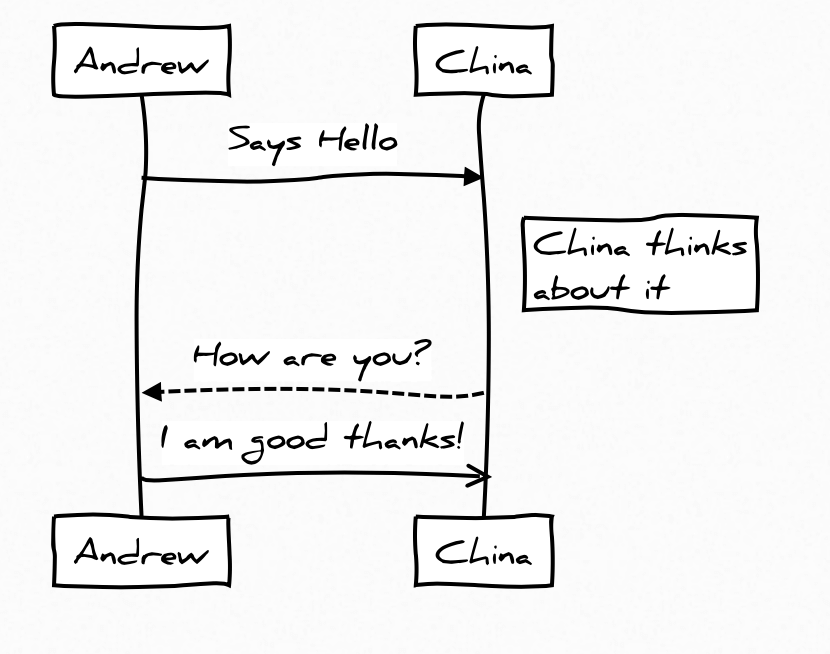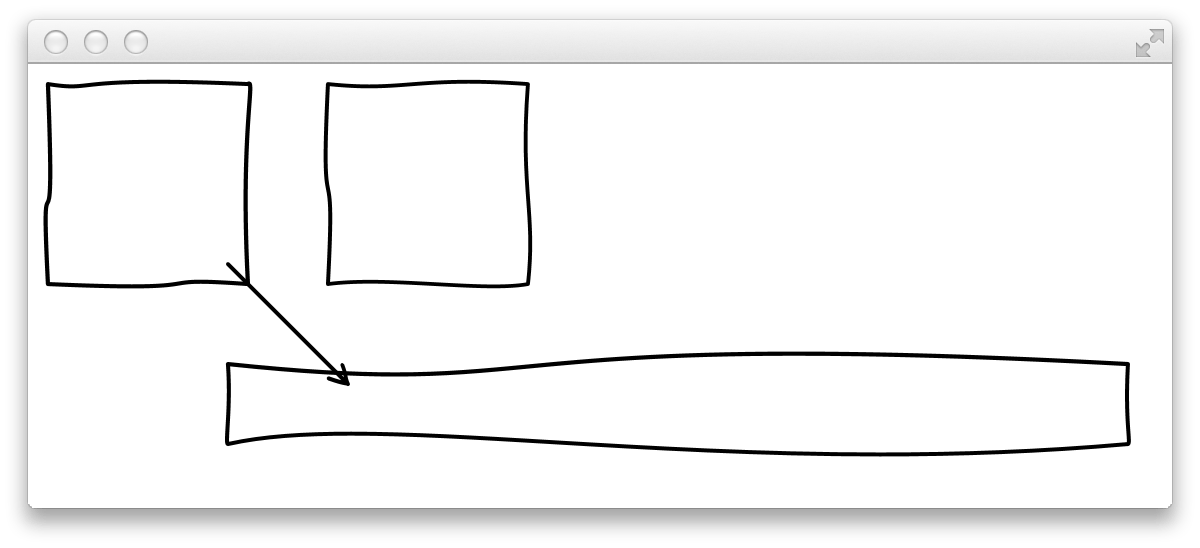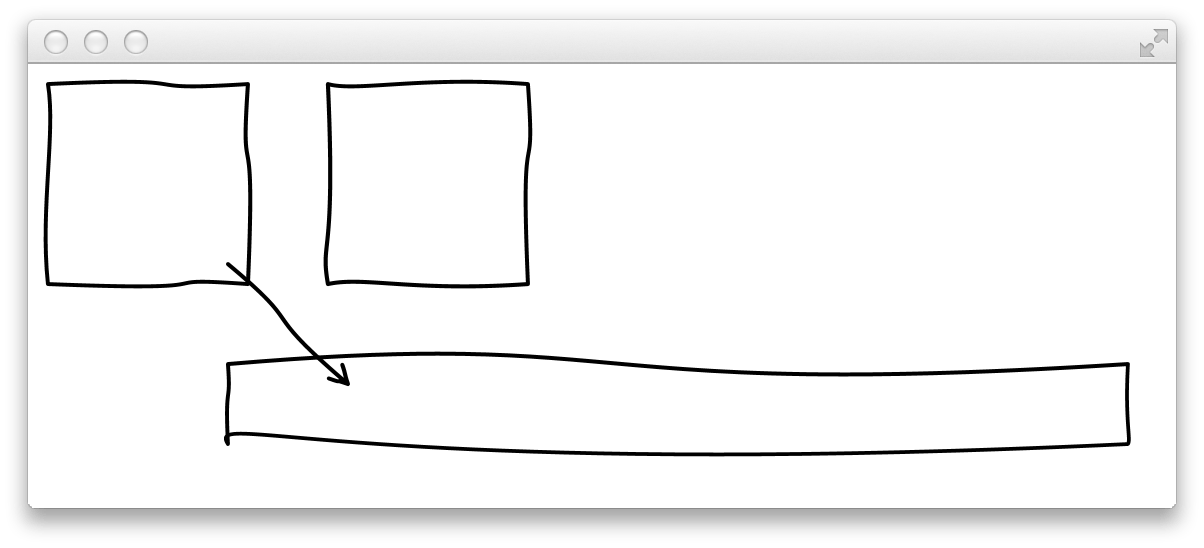EN# Hand drawing effect with JavaFXSome days ago Thierry Wasylczenko blogged about 12 of his favorite JS libs. On of the libs is js-sequence-diagrams that I did know. By using the library you can draw sequence diagrams in a browser. I really like the hand drawn theme of the tool that draw all the diagrams like they are sketched with a pen. Here is an example of the theme:After having a look at the source code of js-sequence-diagrams I found the methods that render all the hand drawn lines. Cubic curves are used here and the control points of the curves are calculated by using random values. These two control points define the bend of the curve as you can see in the following picture:Once I’ve seen this I wanted to try if I can do the same with JavaFX :)

Thankfully JavaFX contains support for cubic curves. The Path shape can contain curves that can be defined by the CubicCurveTo class. By using this class you can create lines and shapes that look like hand drawn onces by using the same algorithm as in js-sequence-diagrams. I created a simple test class that draws some arrows, lines and rectangles by using this algorithm. Here are some results:As you can see in the picture the shapes look different in each of them. This is caused by the random values that are part of the algorithm. If you want to try this here is my one class gist that contains all the code:

``````package com.guigarage.incubator.wobble;

import javafx.application.Application;
import javafx.geometry.Point2D;
import javafx.scene.Group;
import javafx.scene.Scene;
import javafx.scene.paint.Color;
import javafx.scene.shape.*;
import javafx.stage.Stage;

public class WobbleTest extends Application {

public static void main(String... args) {
launch(args);
}

@Override
public void start(Stage primaryStage) throws Exception {

Group group = new Group();
group.getChildren().add(createHandDrawnRect(10, 10, 100, 100, 2, Color.BLACK));
group.getChildren().add(createHandDrawnRect(100, 150, 450, 40, 2, Color.BLACK));
group.getChildren().add(createHandDrawnRect(150, 10, 100, 100, 2, Color.BLACK));

group.getChildren().add(createHandDrawnArrow(100, 100, 160, 160, 2, Color.BLACK));

primaryStage.setScene(new Scene(group));
primaryStage.show();
}

public Shape createHandDrawnRect(double x1, double y1, double w, double h, double strokeWidth, Color color) {
Shape top = createHandDrawnLine(x1, y1, x1 + w, y1, strokeWidth, color);
Shape bottom = createHandDrawnLine(x1, y1 + h, x1 + w, y1 + h, strokeWidth, color);
Shape left = createHandDrawnLine(x1, y1, x1, y1 + h, strokeWidth, color);
Shape right = createHandDrawnLine(x1 + w, y1, x1 + w, y1 + h, strokeWidth, color);
return Shape.union(top, Shape.union(bottom, Shape.union(left, right)));
}

public Shape createHandDrawnArrow(double x1, double y1, double x2, double y2, double strokeWidth, Color color) {
Shape line = createHandDrawnLine(x1, y1, x2, y2, strokeWidth, color);

double arrowlenght = strokeWidth * 5;
double distance = Math.sqrt(Math.pow(x2 -x1, 2) + Math.pow(y2 -y1, 2));
double unrotatedX = x2 + ((x1 - x2) / distance) * arrowlenght;
double unrotatedY = y2 + ((y1 - y2) / distance) * arrowlenght;

Point2D rotated1 = new Point2D(x2 + (unrotatedX - x2)*Math.cos(0.5) - (unrotatedY - y2)*Math.sin(0.5), y2 + (unrotatedX - x2)*Math.sin(0.5) + (unrotatedY - y2)*Math.cos(0.5));
Shape arrowLeft = createHandDrawnLine(x2, y2, rotated1.getX(), rotated1.getY(), strokeWidth, color);

Point2D rotated2 = new Point2D(x2 + (unrotatedX - x2)*Math.cos(-0.5) - (unrotatedY - y2)*Math.sin(-0.5), y2 + (unrotatedX - x2)*Math.sin(-0.5) + (unrotatedY - y2)*Math.cos(-0.5));
Shape arrowRight = createHandDrawnLine(x2, y2, rotated2.getX(), rotated2.getY(), strokeWidth, color);
return Shape.union(line, Shape.union(arrowLeft, arrowRight));
}

public Shape createHandDrawnLine(double x1, double y1, double x2, double y2, double strokeWidth, Color color) {
Point2D startPoint = new Point2D(x1, y1);
Point2D endPoint = new Point2D(x2, y2);

double wobble = Math.sqrt((endPoint.getX() - startPoint.getX()) * (endPoint.getX() - startPoint.getX()) + (endPoint.getY() - startPoint.getY()) * (endPoint.getY() - startPoint.getY())) / 25;

double r1 = Math.random();
double r2 = Math.random();

double xfactor = Math.random() > 0.5 ? wobble : -wobble;
double yfactor = Math.random() > 0.5 ? wobble : -wobble;

Point2D control1 = new Point2D((endPoint.getX() - startPoint.getX()) * r1 + startPoint.getX() + xfactor, (endPoint.getY() - startPoint.getY()) * r1 + startPoint.getY() + yfactor);
Point2D control2 = new Point2D((endPoint.getX() - startPoint.getX()) * r2 + startPoint.getX() - xfactor, (endPoint.getY() - startPoint.getY()) * r2 + startPoint.getY() - yfactor);

MoveTo startMove = new MoveTo(startPoint.getX(), startPoint.getY());
CubicCurveTo curve = new CubicCurveTo(control1.getX(), control1.getY(),
control2.getX(), control2.getY(),
endPoint.getX(), endPoint.getY());

Path path = new Path(startMove, curve);
path.setStrokeLineCap(StrokeLineCap.ROUND);
path.setStroke(color);
path.setStrokeWidth(strokeWidth + (strokeWidth * (Math.random() - 0.5) / 8.0));
path.setStrokeType(StrokeType.CENTERED);
return path;
}
}``````#### Hendrik Ebbers

Hendrik Ebbers is the founder of Open Elements. He is a Java champion, a member of JSR expert groups and a JavaOne rockstar. Hendrik is a member of the Eclipse JakartaEE working group (WG) and the Eclipse Adoptium WG. In addition, Hendrik Ebbers is a member of the Board of Directors of the Eclipse Foundation.Explore

Privacy